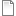Skip to Content

# Polystar

This activity connects number theory and geometry. Your challenge: predict the shape that will result from any combination of angle and distance variables.
Mathematical Experiments with Polystar By Dan Lynn Watt In this activity you can explore the behavior of a script, Polystar, to create geometric designs using two variables, distance and angle. The behavior of the script and specific challenges are described in an attached document, Mathematical Experiments with Polystar.a deceptively simple script for drawing geometric designs
Other Files:Mathematical Experiments with Polystar.pdf